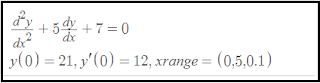## Solving Second Order Differential Equations

In many real-life modeling situations, a differential equation for a variable of interest depends not only on the first derivative but also on the higher ones.

For something more than a second derivative, the question will almost certainly direct you through some special trick that is very specific to the problem at hand.

Also read, Reason behind the huge Demand of Python Developers

For second-order differential equations, you have to know how to deal with them in general. Fortunately, the technology involved is straightforward, and this article guides you through all that you need to know with a useful example!

### Homogeneous Second Order Differential Equations

The first major type of second-order differential equations that you need to learn to solve are the ones that can be written for our dependent variable y and the independent variable t:

Different equations are solved in Python using Scipy.integrate package with the ODEINT function. Another Python package that solves different equations is GEKKO.

Also read, Reason behind the huge Demand of Python Developers

ODEINT requires three inputs:

y = odeint (model, y0, t)

1. model: A function name that returns values based on y.

2. y0: Initial condition.

3. t: Points for the time when the solution should be reported.

The Python code starts importing the required Numpy, Scipy, and Matplotlib packs. Model, initial conditions, and time points are defined as the inclusion in ODEINT arithmetic.

An example of using odeint with the following differential equation.

Example 1:Python Code:
```from matplotlib import pyplot as plt
from scipy.integrate import odeint
import numpy as np
def f(u,x):
return (u,-2*u-2*u+np.cos(2*x))
y0 = [0,0]
xs = np.linspace(1,10,200)
us = odeint(f,y0,xs)
ys = us[:,0]
plt.plot(xs,ys,'-')
plt.plot(xs,ys,'r*')
plt.xlabel('x values')
plt.ylabel('y values')
plt.title('(D**2+2*D+2)y = cos(2*x)')
plt.show()
Output:``````

Also read, Reason behind the huge Demand of Python Developers

Example 2:````Python Code:`
```from matplotlib import pyplot as plt
from scipy.integrate import odeint
import numpy as np
def f(u,x):
return (u,-5 * u-7)
y0 = [21,12]
xs = np.arange(0,5,0.1)
us = odeint(f,y0,xs)
ys = us[:,0]
plt.plot(xs,ys,'-')
plt.plot(xs,ys,'ro')
plt.xlabel('x values')
plt.ylabel('y values')
plt.title('(D**2+ 5*D+7)y=0')
plt.show()```
`Output:`Solving Second Order Differential Equations  ``` Example: 3Python code: from matplotlib import pyplot as plt from scipy.integrate import odeint import numpy as np def f(u,x): return (u,-x**2*np.sin(u)) u0 = [1,0] xs = np.arange(0,3,0.5) us = odeint(f,u0,xs) ys = us[:,0] plt.plot(xs,ys,'-') plt.plot(xs,ys,'ro') plt.xlabel('x values') plt.ylabel('y values') plt.title('(D**2+x**2.sin(y))') plt.show()``` Summary: In this, we discuss how to solving Second-order Differential equations in Python Programming. So, in this very first step is importing the libraries that are necessary. You can also Check this Python Libraries if you read in detail about the Libraries. Matplotlib is a library for Python programming language. Pyplot is a Matplotlib module that is used for plotting. Scipy provides a routine for performing numerical integration. Sometimes a function is complicated to integrate so in that case it can be solved by numerical integration method. The Scipy.integrate used in that case because it contains most of the required function.
```
```